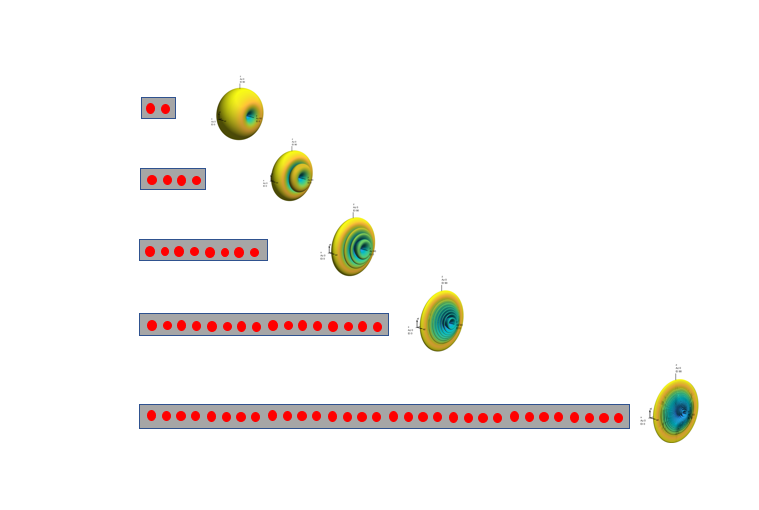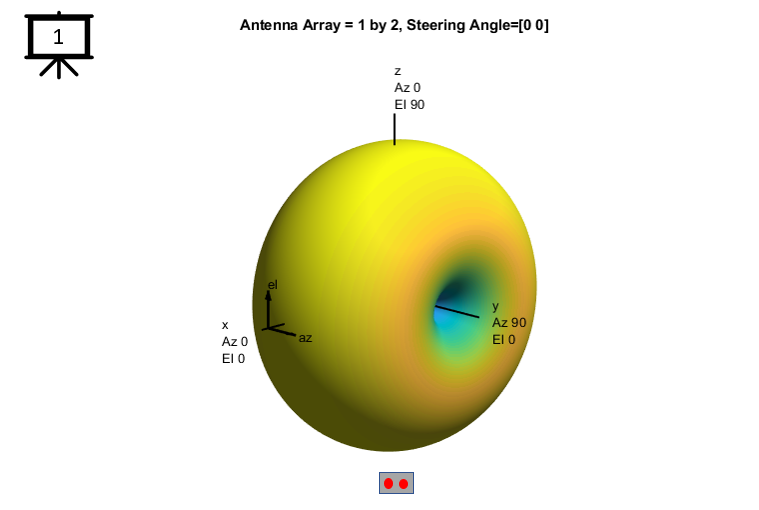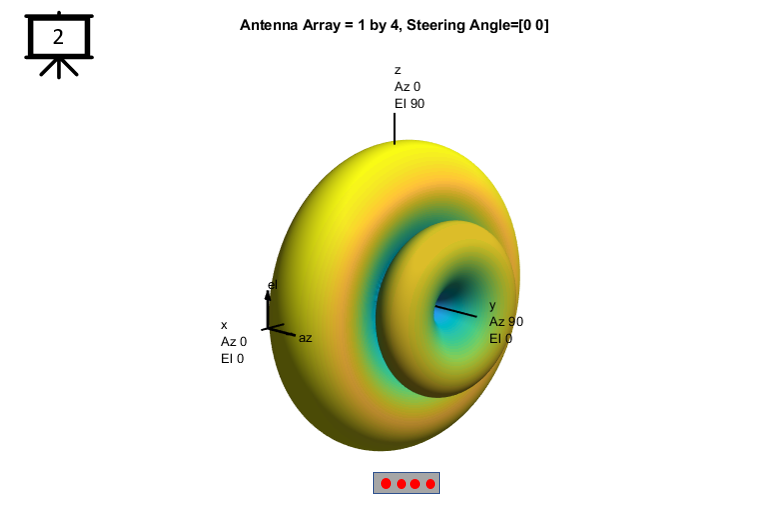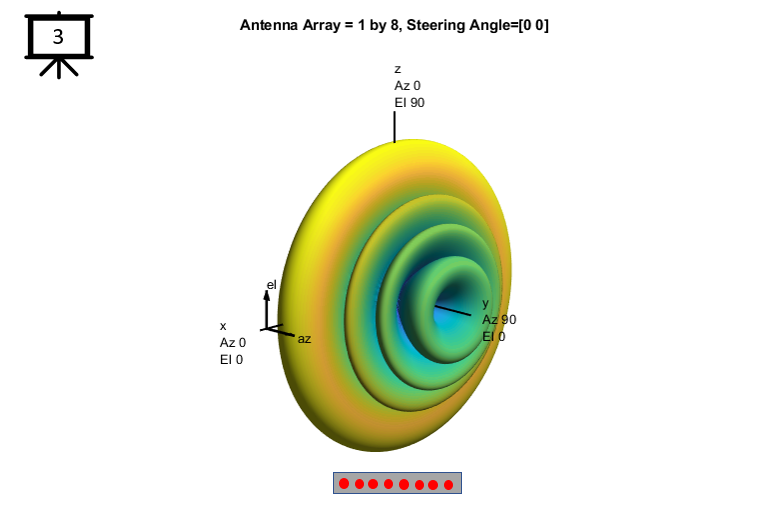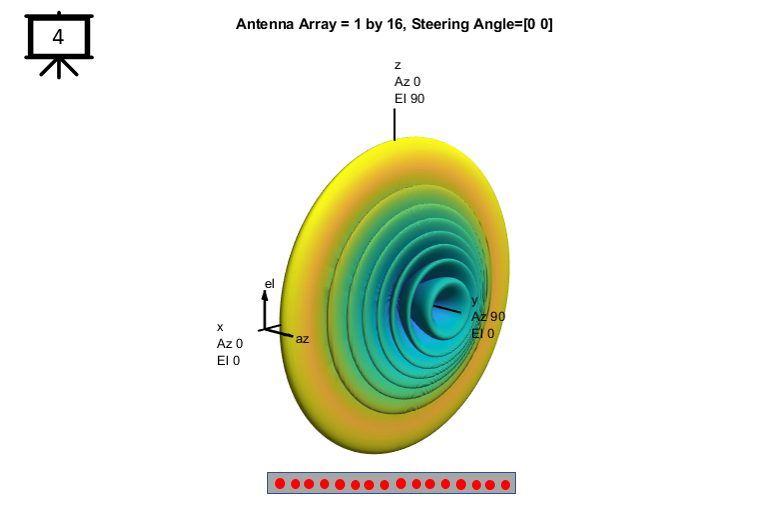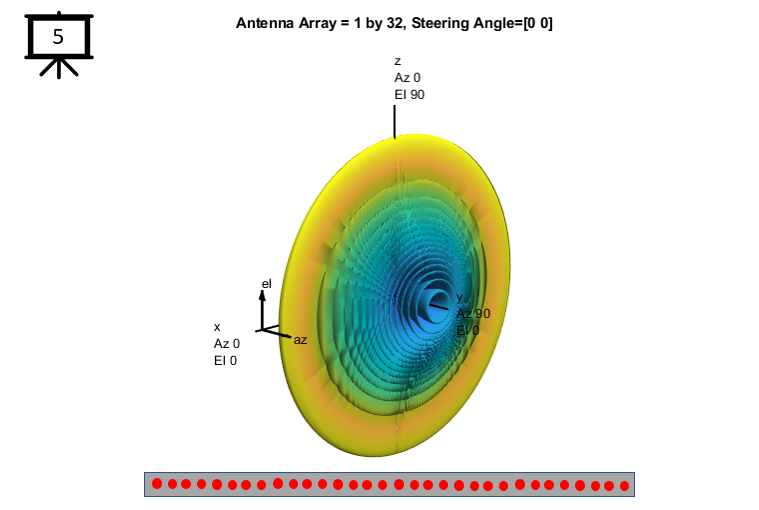Antenna Array - ULA(Uniform Linear Array) : 1 x N     In this tutorial, you will see 3D radiation pattern with various radiation pattern. In all of these array, the number of elements in vertical direction does not change(fixed to 1) and only the number of elements in horizontal direction changes. Pay attention to how the radiation pattern changes as the number of elements in the horizontal direction increases. You would see as the number of elements increases the beam pattern is compressed more in that direction(i.e, the width of beam in vertical direction gets narrower). In this case, the number of elements is fixed to 1 in vertical direction. Threfore, beamforming does not happens in the vertical direction (i.e, the radiation pattern is omnidirectional in vertical direction) and beamforming happens only in horizontal direction since the number of elements increases in that direction.   The principle here is the more antenna elements in a direction, the narrower the beam width gets in that direction. I often use an analagies of a ballon being pressured to help people to build an intuition about the shape of the beam created by the linear array as shown below.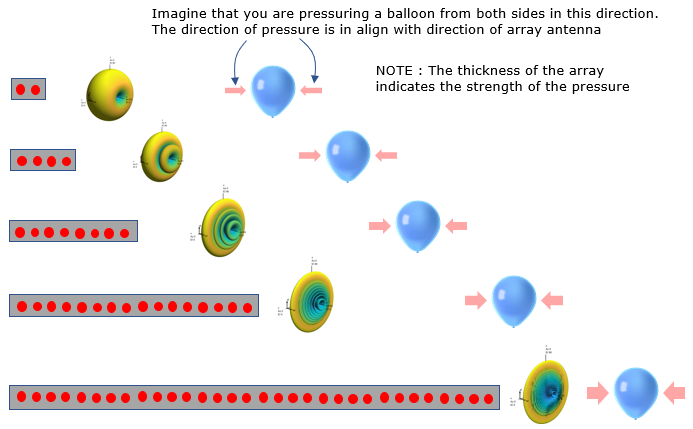NOTE : You can check the cross section of the beam and simple math model for this kind of linear array in this page.     For more theoretical explanation related to these slides, I would suggest you to look into another form of my notes listed below.

 Followings are the code that I wrote in Matlab Phased Array System Toolbox  to creates all the plots shown in this page. You may copy these code and play with these codes. Change variables and try yourself until you get your own intuitive understanding.   < Code 1 >   c = 3e8;        % propagation speed fc = 26e9;      % carrier frequency lambda = c/fc;  % wavelength NoOfTxAntenna = 32   txarray = phased.ULA('NumElements',NoOfTxAntenna,'ElementSpacing',lambda/2);   hFig = figure(1); pattern(txarray,fc,[-180:180],[-90:90],...     'PropagationSpeed',c,...     'CoordinateSystem','polar',...     'Type','powerdb') set(gcf,'color','w');   view(135,15);   sTitle = sprintf("Antenna Array = %d by %d, Steering Angle=[%d %d]",1,NoOfTxAntenna,0,0); title(sTitle); set(hFig,'Position',[300 100 800 700]);     set(hFig,'Position',[300 300 780 300]);